# Draw geometric shapes on images using OpenCV

OpenCV provides many drawing functions to draw geometric shapes and write text on images. Let’s see some of the drawing functions and draw geometric shapes on images using OpenCV.

Some of the drawing functions are :

cv2.line() : Used to draw line on an image.
cv2.rectangle() : Used to draw rectangle on an image.
cv2.circle() : Used to draw circle on an image.
cv2.putText() : Used to write text on image.

To demonstrate uses of the above-mentioned functions we need an image of size 400 X 400 filled with a solid colour (black in this case). Inorder to do this, We can utilize numpy.zeroes function to create the required image.

 `# Python3 program to draw solid-colored ` `# image using numpy.zeroes() function ` `import` `numpy as np ` `import` `cv2 ` ` `  `# Creating a black image with 3 channels ` `# RGB and unsigned int datatype ` `img ``=` `np.zeros((``400``, ``400``, ``3``), dtype ``=` `"uint8"``) ` `cv2.imshow(``'dark'``, img) ` ` `  `# Allows us to see image ` `# untill closed forcefully ` `cv2.waitKey(``0``) ` `cv2.destroyAllWindows() `

Output :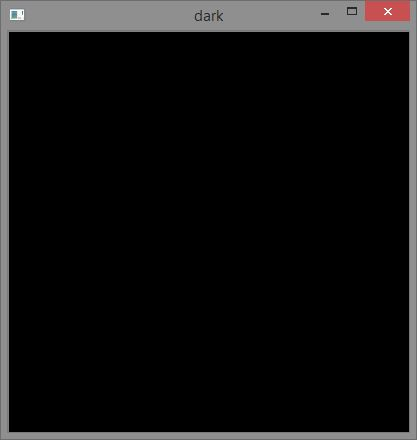Now, let’s draw some geometric shapes on this solid black image.

#### Draw a line :

cv2.line(imageObjectName, (‘start_coordinates’), (‘end_coordinates’), (‘color_in_bgr’), ‘line_thickness’)

 `# Python3 program to draw line ` `# shape on solid image ` `import` `numpy as np ` `import` `cv2 ` ` `  `# Creating a black image with 3 channels ` `# RGB and unsigned int datatype ` `img ``=` `np.zeros((``400``, ``400``, ``3``), dtype ``=` `"uint8"``) ` ` `  `# Creating line ` `cv2.line(img, (``20``, ``160``), (``100``, ``160``), (``0``, ``0``, ``255``), ``10``) ` ` `  `cv2.imshow(``'dark'``, img) ` ` `  `# Allows us to see image ` `# untill closed forcefully ` `cv2.waitKey(``0``) ` `cv2.destroyAllWindows() `

Output :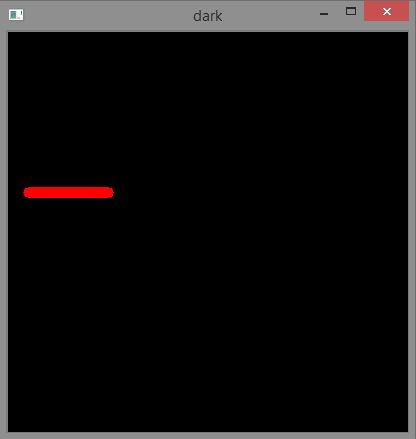#### Draw a rectangle :

cv2.rectangle(imageObjectName, (‘top_left_vertex_coordinates’), (‘lower_right_vertex_coordinates’), (‘stroke_color_in_bgr’), ‘stroke_thickness’)

 `# Python3 program to draw rectangle ` `# shape on solid image ` `import` `numpy as np ` `import` `cv2 ` ` `  `# Creating a black image with 3 ` `# channels RGB and unsigned int datatype ` `img ``=` `np.zeros((``400``, ``400``, ``3``), dtype ``=` `"uint8"``) ` ` `  `# Creating rectangle ` `cv2.rectangle(img, (``30``, ``30``), (``300``, ``200``), (``0``, ``255``, ``0``), ``5``) ` ` `  `cv2.imshow(``'dark'``, img) ` ` `  `# Allows us to see image ` `# untill closed forcefully ` `cv2.waitKey(``0``) ` `cv2.destroyAllWindows() `

Output :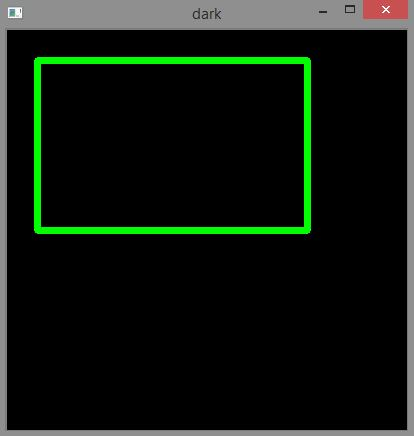#### Draw a Circle :

 `# Python3 program to draw circle ` `# shape on solid image ` `import` `numpy as np ` `import` `cv2 ` ` `  `# Creating a black image with 3 ` `# channels RGB and unsigned int datatype ` `img ``=` `np.zeros((``400``, ``400``, ``3``), dtype ``=` `"uint8"``) ` ` `  `# Creating circle ` `cv2.circle(img, (``200``, ``200``), ``80``, (``255``, ``0``, ``0``), ``3``) ` ` `  `cv2.imshow(``'dark'``, img) ` ` `  `# Allows us to see image ` `# untill closed forcefully ` `cv2.waitKey(``0``) ` `cv2.destroyAllWindows() `

Output :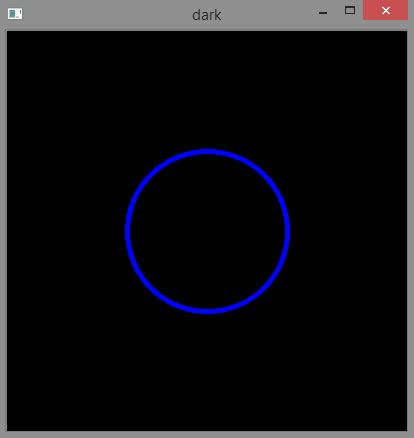#### Writing text :

cv2.putText(imageObjectName, ‘TextContent’, (‘text_starting_point_coordinates’), ‘fontToBeUsed’, ‘font_size’, (‘text_color’, ‘text_thickness’, ‘line_type’)

 `# Python3 program to write  ` `# text on solid image ` `import` `numpy as np ` `import` `cv2 ` ` `  `# Creating a black image with 3 ` `# channels RGB and unsigned int datatype ` `img ``=` `np.zeros((``400``, ``400``, ``3``), dtype ``=` `"uint8"``) ` ` `  `# writing text ` `font ``=` `cv2.FONT_HERSHEY_SIMPLEX ` `cv2.putText(img, ``'GeeksForGeeks'``, (``50``, ``50``), ` `            ``font, ``0.8``, (``0``, ``255``, ``0``), ``2``, cv2.LINE_AA) ` ` `  `cv2.imshow(``'dark'``, img) ` ` `  `# Allows us to see image ` `# untill closed forcefully ` `cv2.waitKey(``0``) ` `cv2.destroyAllWindows() `

Output :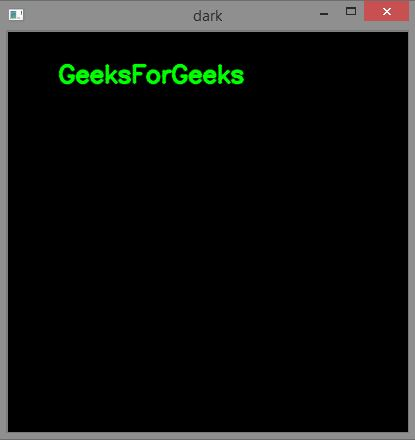Applications of drawing shapes on images :

• Drawing geometrical shapes can help us highlight the particular portions of an image.
• Geometrical shapes like line can help us point or identify particular regions in image.
• Writing text on certain regions of images can add description to that region.

My Personal Notes arrow_drop_upCheck out this Author's contributed articles.

If you like GeeksforGeeks and would like to contribute, you can also write an article using contribute.geeksforgeeks.org or mail your article to contribute@geeksforgeeks.org. See your article appearing on the GeeksforGeeks main page and help other Geeks.

Please Improve this article if you find anything incorrect by clicking on the "Improve Article" button below.

Article Tags :
Practice Tags :

Be the First to upvote.

Please write to us at contribute@geeksforgeeks.org to report any issue with the above content.